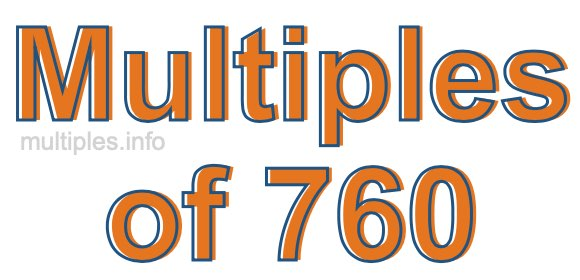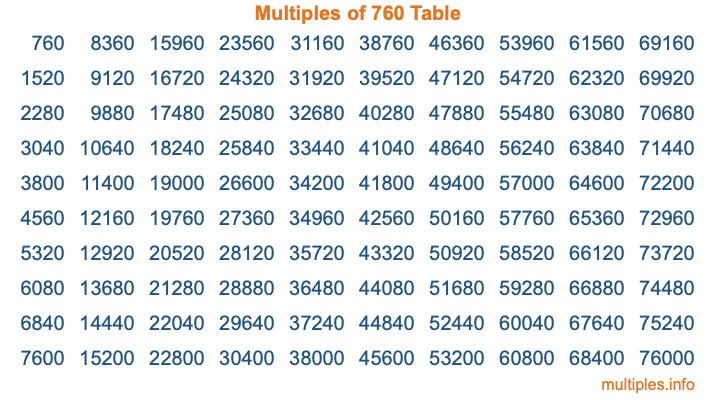Multiples of 760Welcome to the Multiples of 760 page. Here we will first teach you everything you will ever need to know about the multiples of 760, and then give you a study guide summary of everything we taught you to make sure you remember it all. Use this page to look up facts and learn information about the multiples of 760. This page will make you a multiples of seven hundred sixty expert!

Definition of Multiples of 760
Multiples of 760 are all the numbers that when divided by 760 equal an integer. Each of the multiples of 760 are called a multiple. A multiple of 760 is created by multiplying 760 by an integer.

Therefore, to create a list of multiples of 760, you start with 1 multiplied by 760, then 2 multiplied by 760, then 3 multiplied by 760, and so on for as long as you want. Thus, the list of the first five multiples of 760 is 760, 1520, 2280, 3040, and 3800. To see a larger list of multiples of 760, see the printable image of Multiples of 760 further down on this page. We also have a category where you can choose any nth multiple of 760.

Multiples of 760 Checker
The Multiples of 760 Checker below checks to see if any number of your choice is a multiple of 760. In other words, it checks to see if there is any number (integer) that when multiplied by 760 will equal your number. To do that, we divide your number by 760. If the the quotient is an integer, then your number is a multiple of 760.

Is  a multiple of 760?

Least Common Multiple of 760 and ...
A Least Common Multiple (LCM) is the lowest multiple that two or more numbers have in common. This is also called the smallest common multiple or lowest common multiple and is useful to know when you are adding our subtracting fractions. Enter one or more numbers below (760 is already entered) to find the LCM.

Check out our LCM Calculator if you need more details about the Least Common Multiple or if you need the LCM for different numbers for adding and subtraction fractions.

nth Multiple of 760
As we stated above, 760 is the first multiple of 760, 1520 is the second multiple of 760, 2280 is the third multiple of 760, and so on. Enter a number below to find the nth multiple of 760.

th multiple of 760

Multiples of 760 vs Factors of 760
760 is a multiple of 760 and a factor of 760, but that is where the similarities end. All postive multiples of 760 are 760 or greater than 760. All positive factors of 760 are 760 or less than 760.

Below is the beginning list of multiples of 760 and the factors of 760 so you can compare:

Multiples of 760: 760, 1520, 2280, 3040, 3800, etc.

Factors of 760: 1, 2, 4, 5, 8, 10, 19, 20, 38, 40, 76, 95, 152, 190, 380, 760

As you can see, the multiples of 760 are all the numbers that you can divide by 760 to get a whole number. The factors of 760, on the other hand, are all the whole numbers that you can multiply by another whole number to get 760.

It's also interesting to note that if a number (x) is a factor of 760, then 760 will also be a multiple of that number (x).

Multiples of 760 vs Divisors of 760
The divisors of 760 are all the integers that 760 can be divided by evenly. Below is a list of the divisors of 760.

Divisors of 760: 1, 2, 4, 5, 8, 10, 19, 20, 38, 40, 76, 95, 152, 190, 380, 760

The interesting thing to note here is that if you take any multiple of 760 and divide it by a divisor of 760, you will see that the quotient is an integer.

Multiples of 760 Table
Below is an image of the first 100 multiples of 760 in a table. The table is in chronological order, column by column. The first column has the first ten multiples of 760, the second column has the next ten multiples of 760, and so on.The Multiples of 760 Table is also referred to as the 760 Times Table or Times Table of 760. You are welcome to print out our table for your studies.

Negative Multiples of 760
Although not often discussed or needed in math, it is worth mentioning that you can make a list of negative multiples of 760 by multiplying 760 by -1, then by -2, then by -3, and so on, to get the following list of negative multiples of 760:

-760, -1520, -2280, -3040, -3800, etc.

Multiples of 760 Summary
Below is a summary of important Multiples of 760 facts that we have discussed on this page. To retain the knowledge on this page, we recommend that you read through the summary and explain to yourself or a study partner why they hold true.

There are an infinite number of multiples of 760.

A multiple of 760 divided by 760 will equal a whole number.

760 divided by a factor of 760 equals a divisor of 760.

The nth multiple of 760 is n times 760.

The largest factor of 760 is equal to the first positive multiple of 760.

760 is a multiple of every factor of 760.

760 is a multiple of 760.

A multiple of 760 divided by a divisor of 760 equals an integer.

760 divided by a divisor of 760 equals a factor of 760.

Any integer times 760 will equal a multiple of 760.

Multiples of a Number
Here you can get the multiples of another number, all with the same attention to detail as we did for multiples of 760 on this page.

Multiples of
Multiples of 761
Did you find our page about multiples of seven hundred sixty educational? Do you want more knowledge? Check out the multiples of the next number on our list!